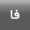[Home ] [Archive]   [ فارسی ]Volume 11, Issue 6 (4-2009)2009, 11(6): 638-646 Back to browse issues page
Comparison of Artificial Neural Network, Logistic Regression and Discriminant Analysis Methods in Prediction of Metabolic Syndrome
Abstract:   (33274 Views)

Abstract

Introduction: Artificial neural networks as a modern modeling method have received considerable attention in recent years. The models are used in prediction and classification in situations where classic statistical models have restricted application when some, or all of their assumptions are met. This study is aimed to compare the ability of neural network models to discriminant analysis and logistic regression models in predicting the metabolic syndrome. Materials & Methods: A total of 347 participants from the cohort of the Tehran Lipid and Glucose Study (TLGS) were studied. The subjects were free of metabolic syndrome at baseling according to the ATPIII criteria. Demographic characteristics, history of coronary artery disease, body mass index, waist, LDL, HDL, total cholesterol, triglycerides, fasting and 2 hours blood sugar, smoking, systolic and diastolic blood pressure were measured at baseline. Incidence of metabolic syndrome after about 3 years of follow up was considered a dependent variable. Logistic regression, discriminant analysis and neural network models were fitted to the data. The ability of the models in predicting metabolic syndrome was compared using ROC analysis and the Kappa statistic, for which, MATLAB software was used. Results: The areas under receiver operating characteristic (ROC) curve for logistic regression, discriminant analysis and artificial neural network models (15: 8: 1) and (15: 10: 10) were estimated as 0. 749, 0. 739, 0. 748 and 0. 890 respectively. Sensitivity of models were calculated as 0. 483, 0. 677, 0. 453 and 0. 863 and their specificity as 0. 857, 0. 660, 0. 910 and 0. 844 respectively. The Kappa statistics for these models were 0. 322, 0. 363, 0. 372 and 0. 712 respectively. Conclusion: Results of this study indicate that artificial neural network models perform better than classic statistical models in predicting the metabolic syndrome.

Keywords: Artificial Neural Network, Logistic Regression, Discriminant Analysis, Metabolic syndrome
Type of Study: Original |